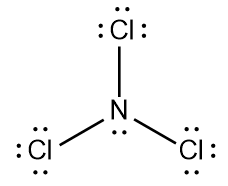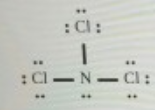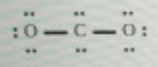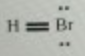# Problem: Decide whether these proposed Lewis structures are reasonable.Proposed Lewis structure Is the proposed Lewis structure reasonable? a) Yes b) No, It has the wrong number of valence electrons The correct number is: ___c) No, t has the right number of valence electrons but doesn't satisty the octet rule. The symbols of the problem atoms are:* ___Proposed Lewis structure Is the proposed Lewis structure reasonable? a) Yes b) No, It has the wrong number of valence electrons The correct number is: ___c) No, t has the right number of valence electrons but doesn't satisty the octet rule. The symbols of the problem atoms are:* ___Proposed Lewis structureIs the proposed Lewis structure reasonable? a) Yes b) No, It has the wrong number of valence electrons The correct number is: ___c) No, t has the right number of valence electrons but doesn't satisty the octet rule. The symbols of the problem atoms are:* ___If two or more atoms of the same element don't satisfy the octet rule, just enter the chemical symbol as many times as necessary. For example, if two oxygen atoms don't satisfy the octet rule, enter "O,O"

###### FREE Expert Solution

For:88% (72 ratings)###### Problem Details

Decide whether these proposed Lewis structures are reasonable.

Proposed Lewis structureIs the proposed Lewis structure reasonable?

a) Yes

b) No, It has the wrong number of valence electrons The correct number is: ___

c) No, t has the right number of valence electrons but doesn't satisty the octet rule. The symbols of the problem atoms are:* ___

Proposed Lewis structureIs the proposed Lewis structure reasonable?

a) Yes

b) No, It has the wrong number of valence electrons The correct number is: ___

c) No, t has the right number of valence electrons but doesn't satisty the octet rule. The symbols of the problem atoms are:* ___

Proposed Lewis structureIs the proposed Lewis structure reasonable?

a) Yes

b) No, It has the wrong number of valence electrons The correct number is: ___

c) No, t has the right number of valence electrons but doesn't satisty the octet rule. The symbols of the problem atoms are:* ___

If two or more atoms of the same element don't satisfy the octet rule, just enter the chemical symbol as many times as necessary. For example, if two oxygen atoms don't satisfy the octet rule, enter "O,O"